# Three reds

What is the probability that when choosing 3 carats from seven carats, all 3 reds will be red?

p =  1.129 %

### Step-by-step explanation: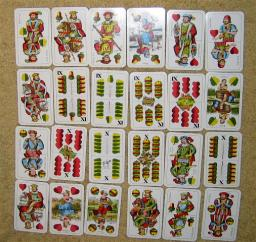Did you find an error or inaccuracy? Feel free to write us. Thank you!Tips to related online calculators
Would you like to compute count of combinations?

## Related math problems and questions:

• CardsFrom a set of 32 cards, we randomly pull out three cards. What is the probability that it will be seven kings and ace?
• Boys and girlsThere are 11 boys and 18 girls in the classroom. Three pupils will answer. What is the probability that two boys will be among them?
• Five-digit numbersHow many different five-digit numbers can be created from the numbers 2,3,5 if the number 2 appears in the number twice and the number 5 also twice?How many ways can we thread 4 red, 5 blue, and 6 yellow beads onto a thread?
• Three colors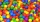Find the probability that 3 balls of the same color will be drawn from fate with 10 white, 10 red, and 10 blue balls.
• Playing cards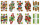From 32 playing cards containing 8 red cards, we choose 4 cards. What is the probability that just 2 will be red?
• A marriedA married couple planned to have 3 children. i. List the possible combinations of the sexes of 3 children. Use B for boy and G for girl. ii. Calculate the probability that all three children would be of the same gender
• Two groupsThe group of 10 girls should be divided into two groups with at least 4 girls in each group. How many ways can this be done?
• STRESSED wordEach letter in STRESSED is printed on identical cards, one letter per card, and assembled in random order. Calculate the probability that the cards spell DESSERTS when assembled.
• Sum or productWhat is the probability that two dice fall will have the sum 7 or product 12?
• All use computerIt is reported that 72% of working women use computers at work. Choose 3 women at random, find the probability that all 3 women use a computer in their jobs.
• Peaches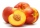There are 20 peaches in the pocket. 3 peaches are rotten. What is the probability that one of the randomly picked two peaches will be just one rotten?
• Bulbs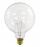The probability that the bulb can operate 4000 hours is 0.3. What is the probability that exactly one of eight bulbs can operate 4000 hours?
• Three students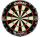Three students independently try to solve the problem. The first student will solve a similar problem with a probability of 0.6, the second student will solve at a probability of 0.55, and the third will solve at a probability of 0.04. The problem is reso
• Chocolates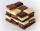In the market we have 3 kinds of chocolates. How many ways can we buy 14 chocolates?
• Families 2Seven hundred twenty-nine families are having six children each. The probability of a girl is 1/3, and the probability of a boy is 2/3. Find the number of families having two girls and four boys.
• BallsFrom the urn in which are 7 white balls and 17 red, gradually drag 3-times without replacement. What is the probability that pulls balls are in order: red red red?